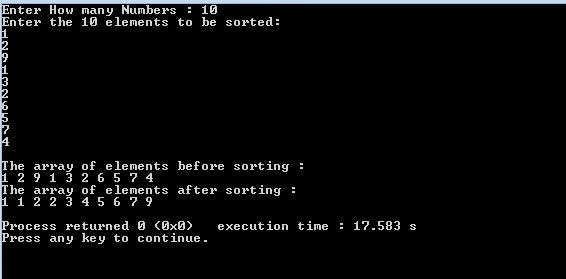## Bucket Sort:

Like as Quick sort and insertion sort, there is another sort called Bucket sort. Bucket sort assumes that the input is drawn from a uniform distribution. Bucket sort runs in linear time on the average which is 0(n). It assumes that the input is generated by a random process that distributes elements uniformly over the interval [0, 1].

#### Bucket sort works as follows:

•     Set up an array of initially empty buckets
•     Go over the original array, putting each object in its bucket.
•     Sort each non-empty bucket.
•     Visit the buckets in order and put all elements back into the original array.
Suppose we need to sort an array of positive integers.
A= {3,4,11,0,2,8,5,9,1,5,6,2,8,0,2,4,1,3,10,4,7,9}.
A bucket sort works as follows: create an array of size 11. Then, go through the input array and place integer 3 into a second array at index 3, integer 11 at index 11 and so on. We will end up with a sorted list in the second array.

#### Array A:

Moving through the array we increment counters:

#### Array B:

Next, we simply read off the number of each occurrence :

#### Output:Output Screen
We can say Bucket sort is asymptotically fast (O(n) when distribution is uniform) . It is a simple to code and good for a rough sort.

## Let's try to print some of the following pattern in java:

We can play with * and print them in many ways, many style. Let's see some awesome pattern of stars and try to do this by computer.
At first try yourself, then go to see code.
Pattern 1: